# KSEEB SSLC Class 10 Maths Solutions Chapter 3 Pair of Linear Equations in Two Variables Ex 3.4

KSEEB SSLC Class 10 Maths Solutions Chapter 3 Pair of Linear Equations in Two Variables Ex 3.4 are part of KSEEB SSLC Class 10 Maths Solutions. Here we have given Karnataka SSLC Class 10 Maths Solutions Chapter 3 Pair of Linear Equations in Two Variables Exercise 3.4.

## Karnataka SSLC Class 10 Maths Solutions Chapter 3 Pair of Linear Equations in Two Variables Exercise 3.4

Question 1.
Solve the following pair of linear equations by the elimination method and the substitution method :
(i) x + y = 5 and 2x – 3y = 4
(ii) 3x + 4y =10 and 2x – 2y = 2
(iii) 3x – 5y – 4 = 0 and 9x = 2y + 7
(iv) $$\frac{x}{2}+\frac{2 y}{3}=-1 \quad \text { and } \quad x-\frac{y}{3}=3$$
Solution:
(i) x + y = 5 and 2x – 3y = 4
x + y = 5 ………….. (i)
2x – 3y = 4 ………… (ii)
Multiplying eqn. (i) by 3
3x + 3y = 15 ………….. (iii)
By adding eqn. (ii) to eqn. (iii) ‘y’ is eliminated.∴ $$x=\frac{19}{5}$$
Substituting the value of ‘x’ in eqn. (i),
x + y = 5
$$\frac{19}{5}+y=5$$
$$y=\frac{5}{1}-\frac{19}{5}$$
$$=\frac{25-19}{5}$$
$$\quad y=\frac{6}{5}$$
$$\quad x=\frac{19}{5} y=\frac{6}{5}$$

(ii) 3x + 4y = 10 and 2x – 2y = 2
3x + 4y = 10 ……….. (i)
2x – 2y = 2 …………. (ii)
Multiplying eqn. (ii) by 2,
4x – 4y = 4 ………….. (iii)
Adding eqn. (i) to eqn. (ii),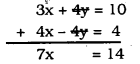∴ $$\quad x=\frac{14}{7}=2$$
Substituting the value of ‘x’ in eqn. (i),
3x + 4y = 10
3 × 2 + 4y = 10
6 + 4y = 10
4y = 10 – 6
4y = 4
∴ $$\mathrm{y}=\frac{4}{4}=1$$
∴ x = 2, y = 1.

(iii) 3x – 5y – 4 = 0 and 9x = 2y + 7
3x – 5y – 4 = 0 ⇒ 3x – 5y = 4 ……… (i)
9x = 2y + 7 ⇒ 9x – 2y = 7 ………. (ii)
Multiplying eqn. (i) by 3,
9x – 15y = 12 …………. (iii)
Subtracting eqn. (iii) from eqn. (ii)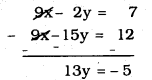∴ $$\quad y=\frac{-5}{13}$$
Substituting the value of ‘y’ in eqn. (i),
3x – 5y = 4
$$3 x-5\left(\frac{-5}{13}\right)=4$$
$$3 x+\frac{25}{13}=4 \quad \text { OR } \quad 3 x=4-\frac{25}{13}$$
$$3 x=\frac{52-25}{13}=\frac{27}{13}$$
$$3 x=\frac{27}{13}$$
∴ $$\quad x=\frac{27}{13} \times \frac{1}{3}$$
∴ $$\quad x=\frac{9}{13}$$
∴ $$\quad x=\frac{9}{13} \quad y=\frac{-5}{13}$$

(iv) $$\frac{x}{2}+\frac{2 y}{3}=-1 \text { and } x-\frac{y}{3}=3$$
$$\frac{x}{2}+\frac{2 y}{3}=-1 \quad \frac{x}{1}-\frac{y}{3}=3$$
$$\frac{3 x+4 y}{6}=-1 \quad \frac{3 x-y}{3}=3$$
3x + 4y = -6 ……….. (i) 3x – y = 9 …………… (ii)
Subtracting eqn. (ii) from eqn. (i),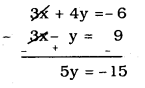$$y=\frac{-15}{3}=-3$$
y = -3
Substituting the value of ‘y’ in eqn. (i),
3x + 4y = -6
3x + 4 (-3) = -6
3x – 12 = -6
3x = -6 + 12
3x = 6
∴ $$\quad x=\frac{6}{3} \quad=2$$
∴ x = 2, y = 3

Question 2.
Form the pair of linear equations in the following problems, and find their solutions (if they exist) by the elimination method:

(i) If we add 1 to the numerator and subtract 1 from the denominator, a fraction reduces to 1. It becomes $$\frac{1}{2}$$ if we only add 1 to the denominator. What is the fraction ?
Solution:
Let the fraction be $$\frac{x}{y}$$, Here numerator is x and denominator is y.
∴ $$\quad \frac{\mathrm{x}+1}{\mathrm{y}-1}=1$$
x + 1 = y – 1
x – y = -1 – 1
x – y = -2 ……….. (i)
$$\frac{x}{y+1}=\frac{1}{2}$$
2 × x = y + 1
2x = y + 1
2x – y = 1 ………. (ii)
Subtracting eqn. (ii) from eqn. (i),∴ x = 3
Substituting the value of ‘x’ in eqn. (i),
x – y = -2
3 – y = -2
-y = -2 -3
-y = -5
∴ y = 5
∴ Fraction is $$\frac{x}{y}=\frac{3}{5}$$

(ii) Five years ago, Nuri was thrice as old as Sonu. Ten years later, Nuri will be twice as old as Sonu. How old are Nuri and Sonu ?
Solution:
Present age of Nuri is ‘x’
Present age of Sonu is ‘y’
Five years ago, age of Nuri is x – 5
Five years ago, age of Sonu is y – 5
then, x – 5 = 3(y – 5)
x – 5 = 3y – 15
x – 3y = -15 + 5
x – 3y = -10 ……….. (i)
After 10 years, age of Nuri will be x + 10
After 10 years, age of Sonu will be y + 10
then, x +10 = 2(y + 10)
x + 10 = 2y + 20
x – 2y = 20 – 10
x – 2y = 10 …………. (ii)
Subtracting eqn. (ii) in eqn. (i),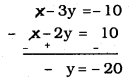∴ y = 20
Substituting the value of ‘y’ in eqn. (ii),
x – 2y = 10
x – 2(20) = 10
x – 40 = 10
x = 10 + 40
x = 50
∴ Present age of Nuri, x = 50
Present age of Sonu, y = 20.

(iii) The sum of the digits of a two-digit number is 9. Also, nine times this number is twice the number obtained by reversing the order of the digits. Find the number.
Solution:
Let the two digit number is 10x + y.
Sum of the digits of to digit number is
x + y = 9 ………….. (i)
Number obtained by reversing the order of the digits, we get 10y + x.
Twicing the 10y + x, it is nine times of first number.
∴ 2(10y + x) = 9(10x + 4)
20y + 2x = 90x + 9y
2x – 90x + 20y – 9y = 0
-88x + 11y = 0
88x – 11y = 0
8x – y = 0 ………….. (ii)
By adding eqn. (i) to eqn. (ii),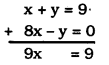∴ $$x=\frac{9}{9}=1$$
Substituting the value of ‘x’ in eqn. (i),
x + y = 9
1 + y = 9
y = 9 – 1
y = 8
∴ x = 1, y = 8
∴ Two digit number = 10x + y
= 10 × 1 + 8
= 10 + 8
=18.

(iv) Meena went to a bank to withdraw Rs. 2000. She asked the cashier to give her Rs. 50 and Rs. 100 notes only. Meena got 25 notes in all. Find how many notes of Rs. 50 and Rs. 100 she received.
Solution:
Let the Rs. 50 notes be ‘x’
Rs. 100 notes b ‘y’
x + y = 25 …………. (i)
x + 2y = 40 …………. (ii)
Subtracting eqn. (ii) from eqn. (i),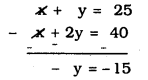∴ y = 15
Substituting the value of ‘y’ in eqn. (i)
x + y = 25
x+ 15 = 25
x = 25 – 15
∴ x = 10
∴ x = 10, y = 15
∴ Rs. 50’s notes are 10 and
Rs. 100’s notes are 15.

(v) A lending library has a fixed charge for the first three days and an additional charge for each day thereafter. Saritha paid Rs. 27 for a book kept for seven days, while Susy paid Rs. 21 for the book she kept for five days. Find the fixed charge and the charge for each extra day.
Solution:
Fixed charge be Rs. x.
x + 4y = 27 ………. (i)
x + 2y = 21 ………. (ii)
Subtracting Eqn. (ii) from eqn. (i),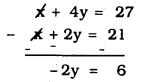∴ $$\quad y=\frac{6}{2} \quad=3$$
Substituting the value of ‘y’ in eqn. (i)
x + 4y = 27
x + 4(3) = 27
x + 12 = 27
∴ x = 27 – 12
∴ x = 15
∴ Fixed charge, x = Rs. 15.
Additional charge, y = Rs. 3.

We hope the given KSEEB SSLC Class 10 Maths Solutions Chapter 3 Pair of Linear Equations in Two Variables Ex 3.4 will help you. If you have any query regarding Karnataka Board SSLC Class 10 Maths Solutions Chapter 3 Pair of Linear Equations in Two Variables Exercise 3.4, drop a comment below and we will get back to you at the earliest.

error: Content is protected !!